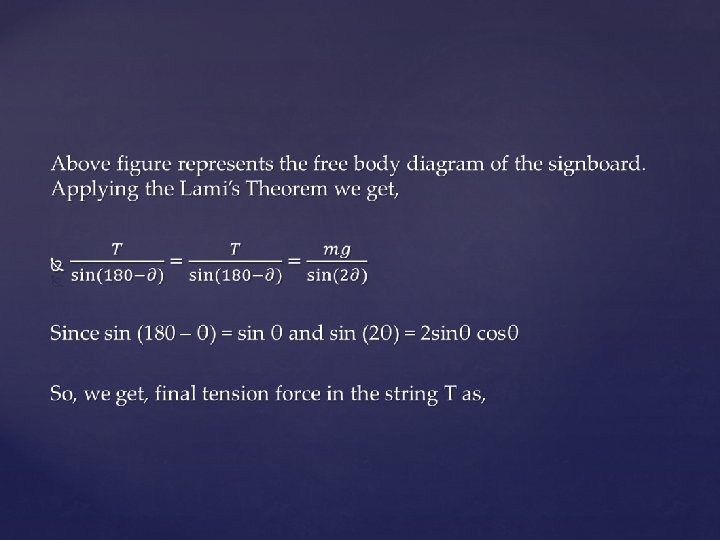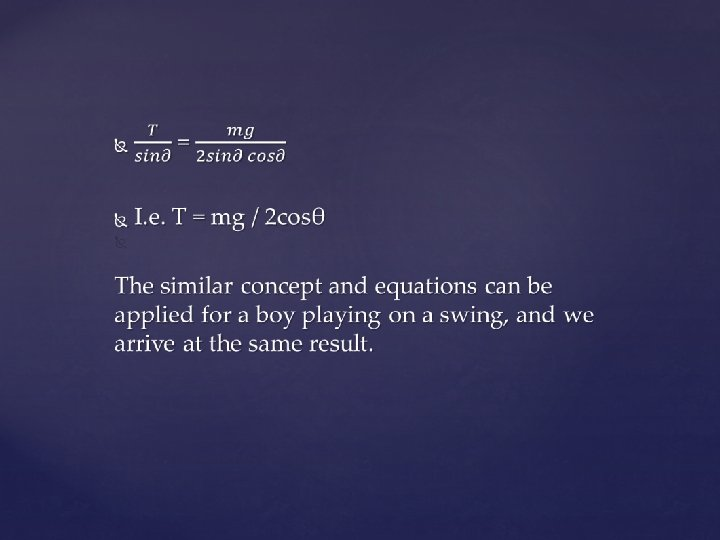# LEMIs Theorem Lamis Theorem Statement Lamis theorem is

• Slides: 8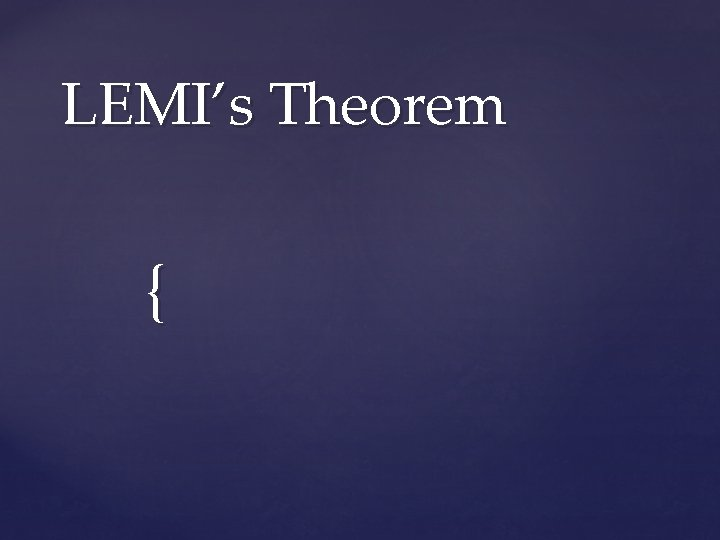LEMI’s Theorem {Lami’s Theorem Statement: Lami's theorem is an equation relating the magnitudes of three coplanar, concurrent and non-collinear forces, which keeps an object in static equilibrium, with the angles directly opposite to the corresponding forces.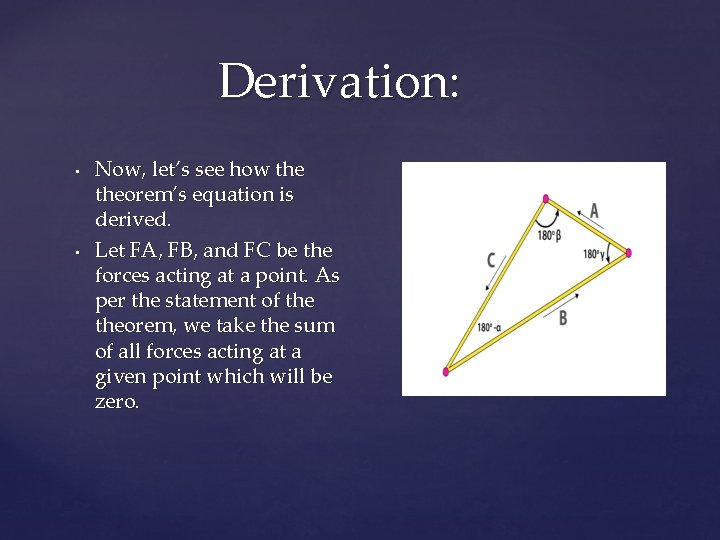Derivation: • • Now, let’s see how theorem’s equation is derived. Let FA, FB, and FC be the forces acting at a point. As per the statement of theorem, we take the sum of all forces acting at a given point which will be zero.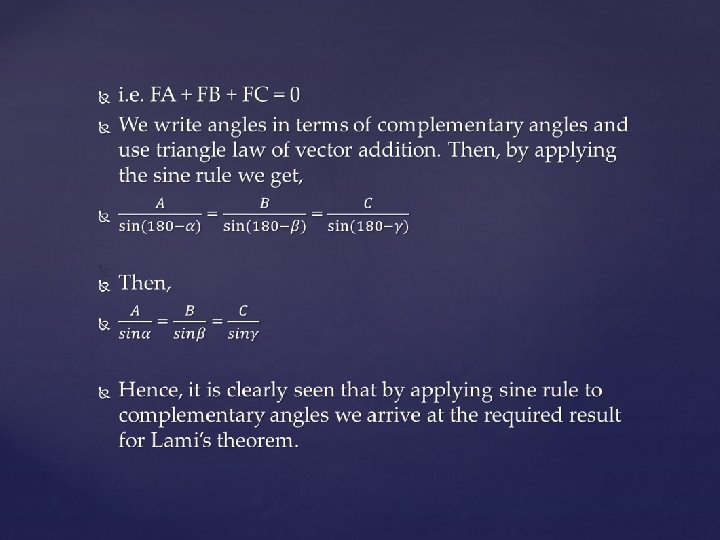Examples: Let 45 degrees be the angle made by the strings with the signboard having a mass of 6 kg, then what is the value of the tension T in both the strings? Given, m = 6 kg, g = 9. 8 m/s², �� = 45 degrees Using the derived formula from example 1, we get, T = mg / 2 cosθ i. e. T = 6 x 9. 8 / 2 cos 45 = 41. 6 N So, the tension in both the strings to hold the signboard exactly horizontal is 41. 6 N.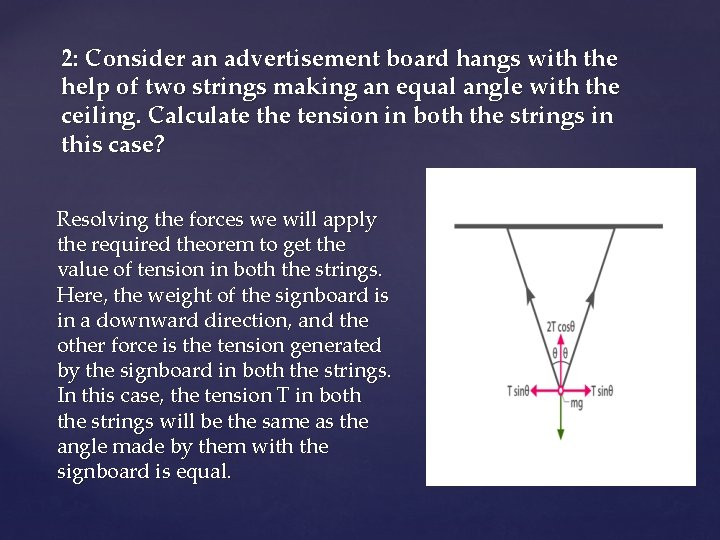2: Consider an advertisement board hangs with the help of two strings making an equal angle with the ceiling. Calculate the tension in both the strings in this case? Resolving the forces we will apply the required theorem to get the value of tension in both the strings. Here, the weight of the signboard is in a downward direction, and the other force is the tension generated by the signboard in both the strings. In this case, the tension T in both the strings will be the same as the angle made by them with the signboard is equal.